# RD Sharma Solutions for Class 11 Chapter 23 - The Straight Lines Exercise 23.15

Here, we shall discuss the concept based on the distance of a point from a line. RD Sharma Solutions for Class 11 pdf can be downloaded effortlessly by students to score good marks in their board examination. The expert faculty team at BYJU’S have solved and prepared the solutions for the exercise wise problems after conducting wide research on each concept. Students can solve problems from the RD Sharma textbook by referring to the solutions to self analyse their weaknesses. The pdf of RD Sharma Class 11 Maths Solutions can be easily downloaded from the links provided below and can be used for future reference as well.

## Download the pdf of RD Sharma Solutions for Class 11 Maths Exercise 23.15 Chapter 23 – The Straight Lines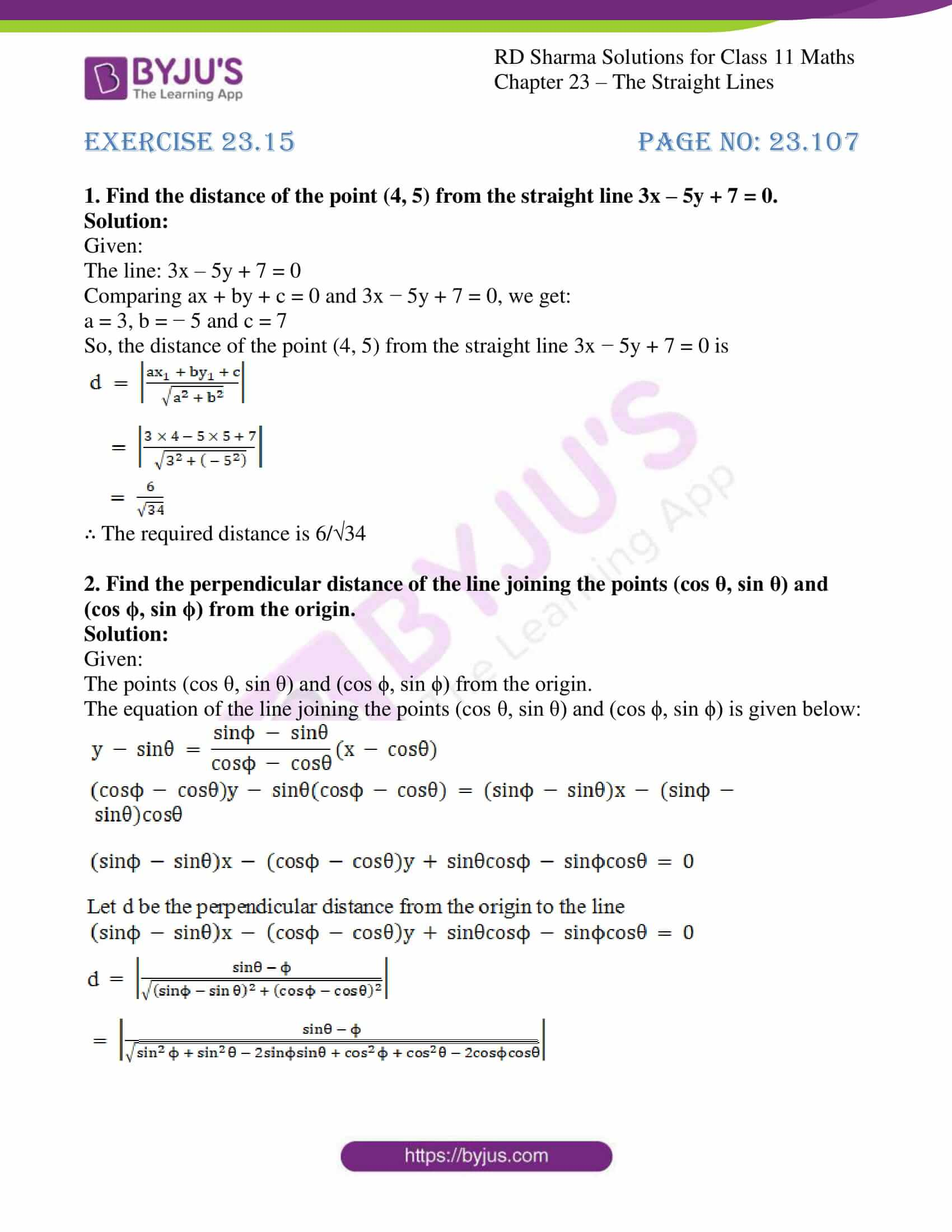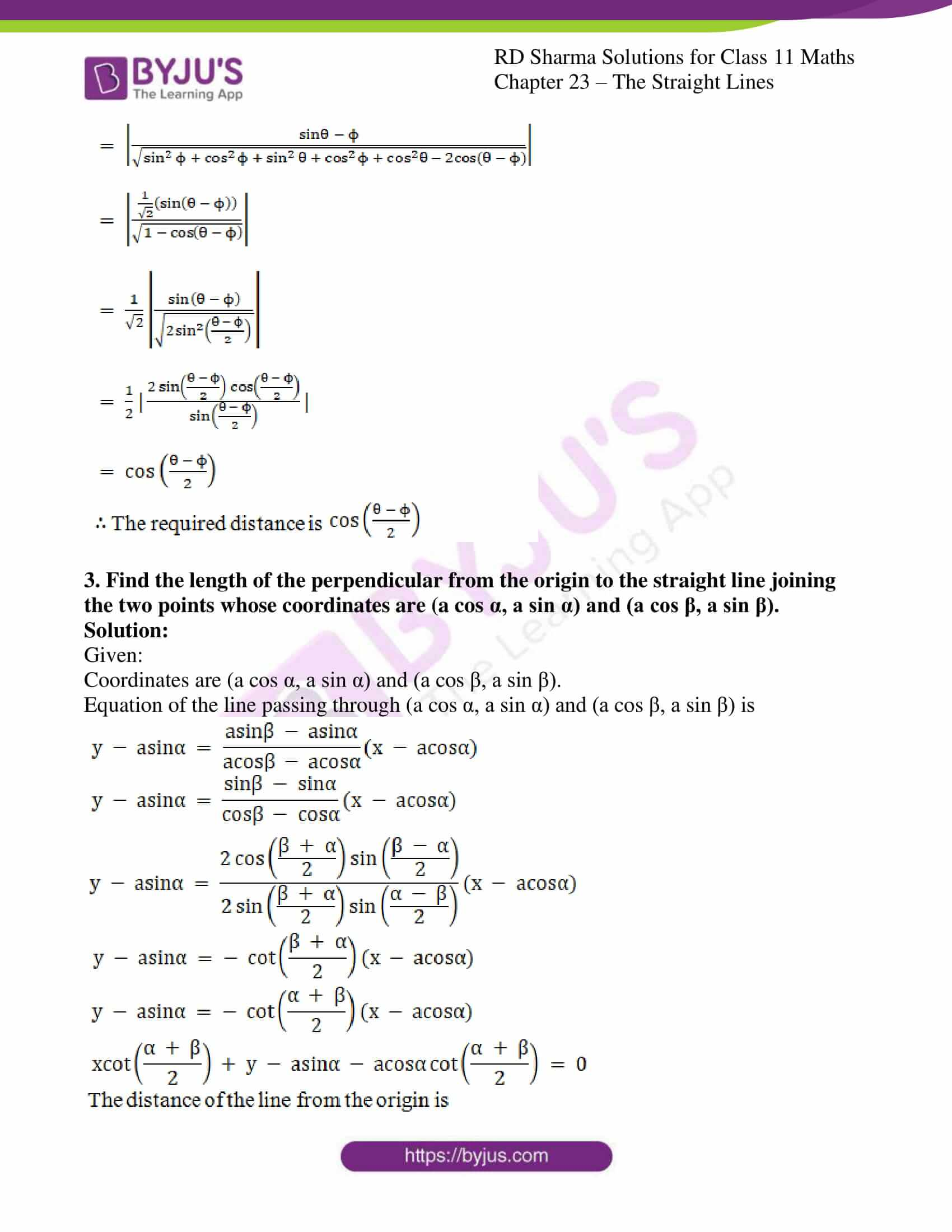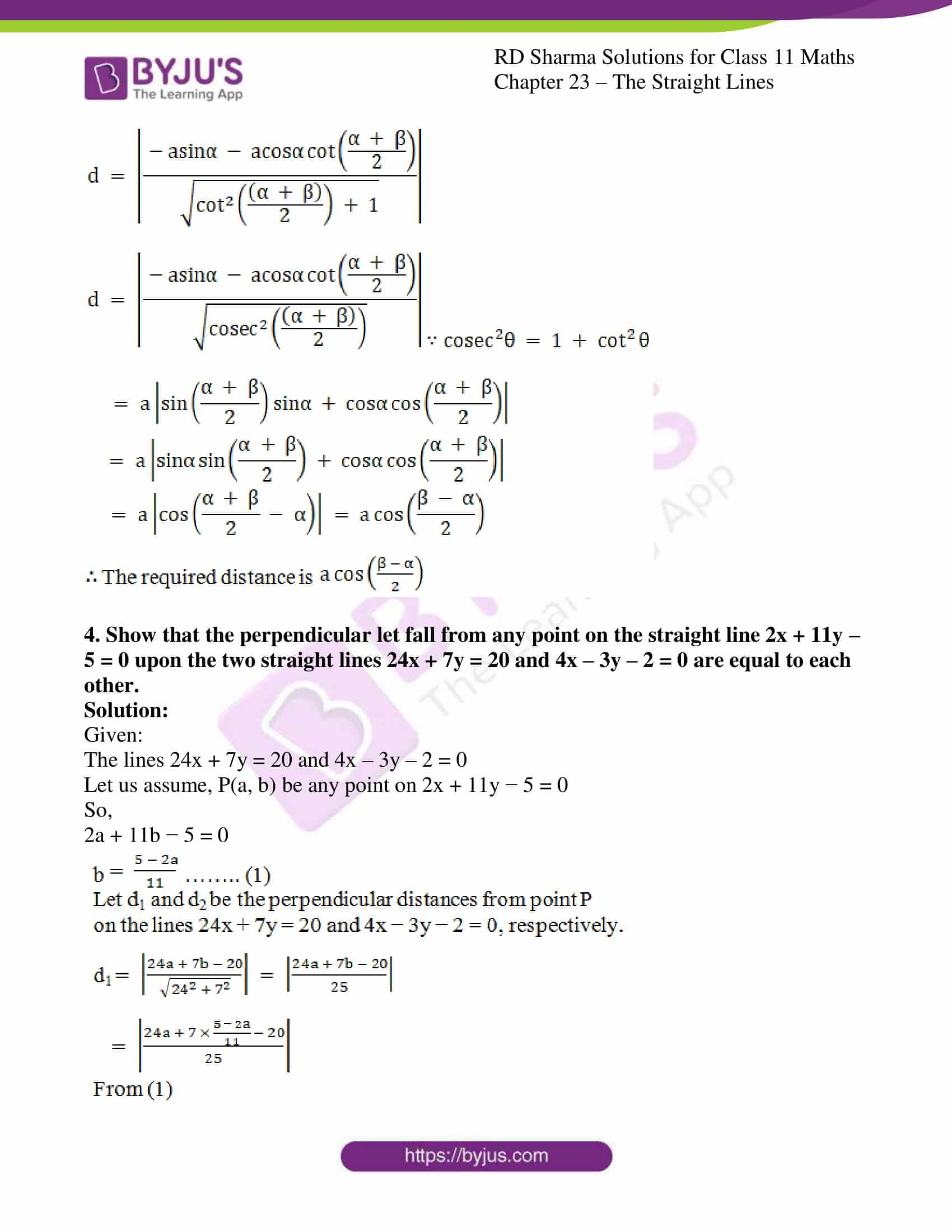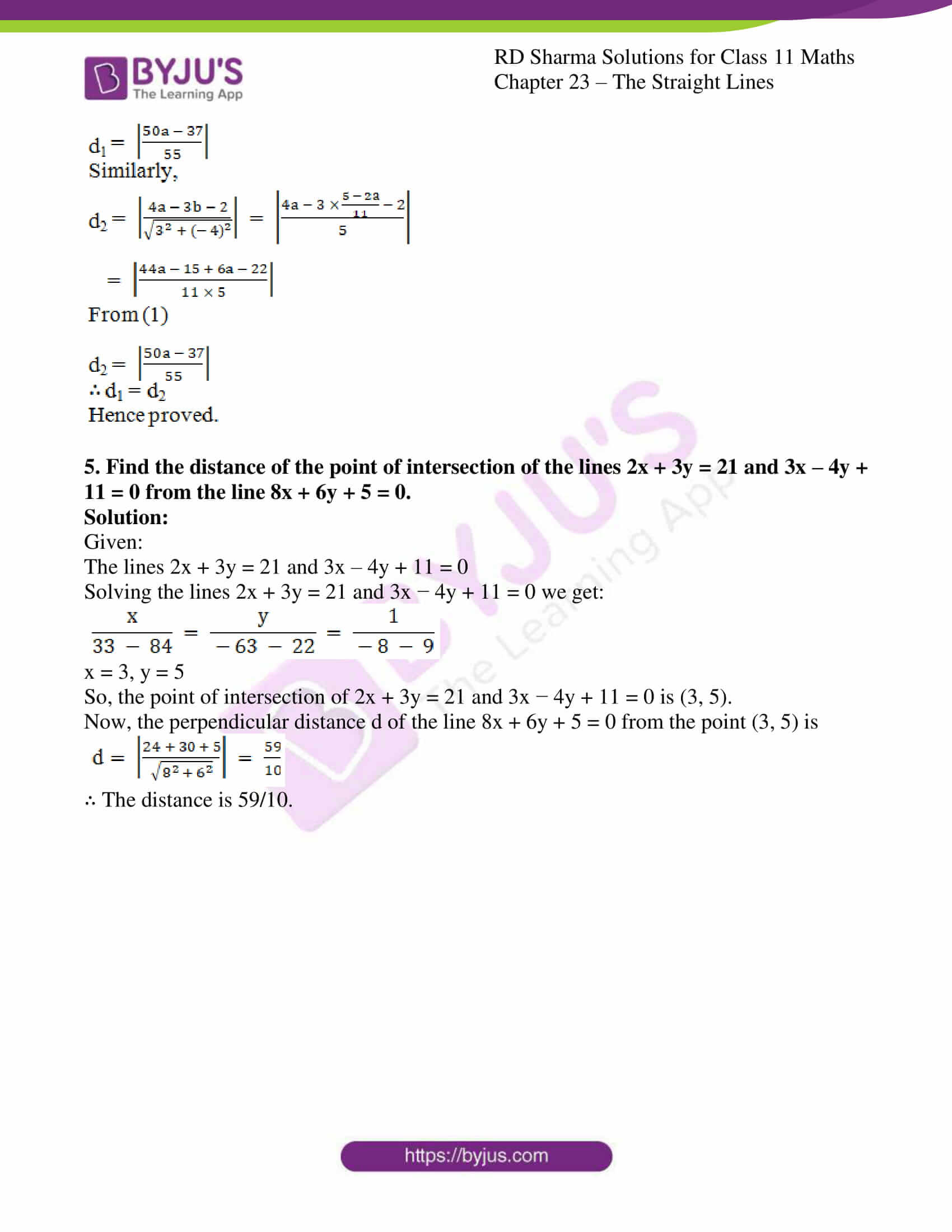### Access answers to RD Sharma Solutions for Class 11 Maths Exercise 23.15 Chapter 23 – The Straight Lines

#### EXERCISE 23.15 PAGE NO: 23.107

1. Find the distance of the point (4, 5) from the straight line 3x – 5y + 7 = 0.

Solution:

Given:

The line: 3x – 5y + 7 = 0

Comparing ax + by + c = 0 and 3x − 5y + 7 = 0, we get:

a = 3, b = − 5 and c = 7

So, the distance of the point (4, 5) from the straight line 3x − 5y + 7 = 0 is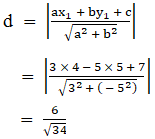∴ The required distance is 6/34

2. Find the perpendicular distance of the line joining the points (cos θ, sin θ) and (cos ϕ, sin ϕ) from the origin.

Solution:

Given:

The points (cos θ, sin θ) and (cos ϕ, sin ϕ) from the origin.

The equation of the line joining the points (cos θ, sin θ) and (cos ϕ, sin ϕ) is given below: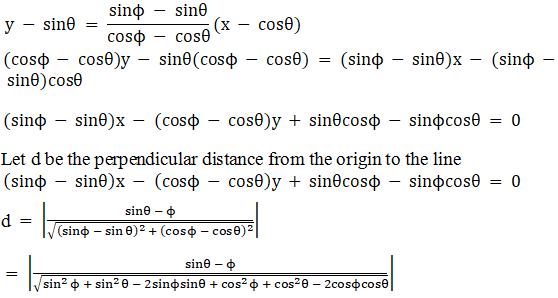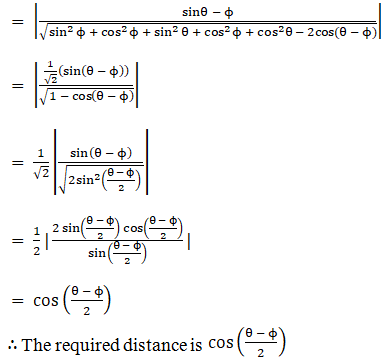3. Find the length of the perpendicular from the origin to the straight line joining the two points whose coordinates are (a cos α, a sin α) and (a cos β, a sin β).

Solution:

Given:

Coordinates are (a cos α, a sin α) and (a cos β, a sin β).

Equation of the line passing through (a cos α, a sin α) and (a cos β, a sin β) is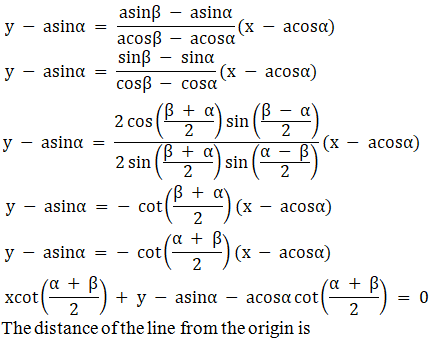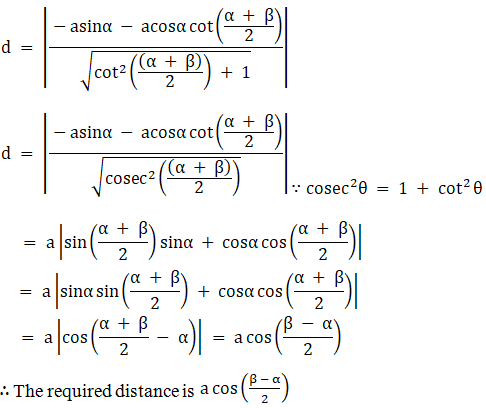4. Show that the perpendicular let fall from any point on the straight line 2x + 11y – 5 = 0 upon the two straight lines 24x + 7y = 20 and 4x – 3y – 2 = 0 are equal to each other.

Solution:

Given:

The lines 24x + 7y = 20 and 4x – 3y – 2 = 0

Let us assume, P(a, b) be any point on 2x + 11y − 5 = 0

So,

2a + 11b − 5 = 0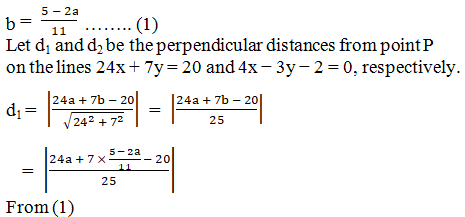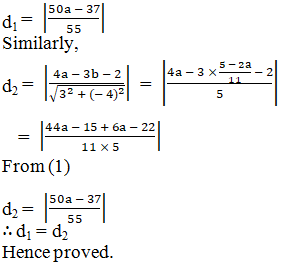5. Find the distance of the point of intersection of the lines 2x + 3y = 21 and 3x – 4y + 11 = 0 from the line 8x + 6y + 5 = 0.

Solution:

Given:

The lines 2x + 3y = 21 and 3x – 4y + 11 = 0

Solving the lines 2x + 3y = 21 and 3x − 4y + 11 = 0 we get: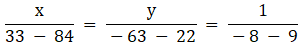x = 3, y = 5

So, the point of intersection of 2x + 3y = 21 and 3x − 4y + 11 = 0 is (3, 5).

Now, the perpendicular distance d of the line 8x + 6y + 5 = 0 from the point (3, 5) is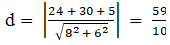∴ The distance is 59/10.